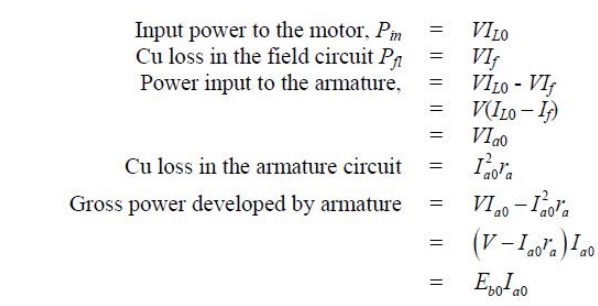Home | | Electrical Machines I | SwinburneŌĆÖs Test

# SwinburneŌĆÖs Test

For a d.c shunt motor change of speed from no load to full load is quite small. Therefore, mechanical loss can be assumed to remain same from no load to full load. Also if field current is held constant during loading, the core loss too can be assumed to remain same.

SwinburneŌĆÖs Test

For a d.c shunt motor change of speed from no load to full load is quite small. Therefore, mechanical loss can be assumed to remain same from no load to full load. Also if field current is held constant during loading, the core loss too can be assumed to remain same.

In this test, the motor is run at rated speed under no load condition at rated voltage. The current drawn from the supply IL0 and the field current If are recorded (figure 40.3). Now we note that:Since the motor is operating under no load condition, net mechanical output power is zero. Hence the gross power developed by the armature must supply the core loss and friction & windage losses of the motor. Therefore,Since, both Pcore and Pfriction for a shunt motor remains practically constant from no load to full load, the sum of these losses is called constant rotational loss i.e.,In the Swinburne's test, the constant rotational loss comprising of core and friction loss is estimated from the above equation.

After knowing the value of Prot from the Swinburne's test, we can fairly estimate the efficiency of the motor at any loading condition. Let the motor be loaded such that new current drawn from the supply is IL and the new armature current is Ia as shown in figure 40.4. To estimate the efficiency of the loaded motor we proceed as follows:The estimated value of Prot obtained from SwinburneŌĆÖs test can also be used to estimate the efficiency of the shunt machine operating as a generator. In figure 40.5 is shown to deliver a load current IL to a load resistor RL. In this case output power being known, it is easier to add the losses to estimate the input mechanical power.